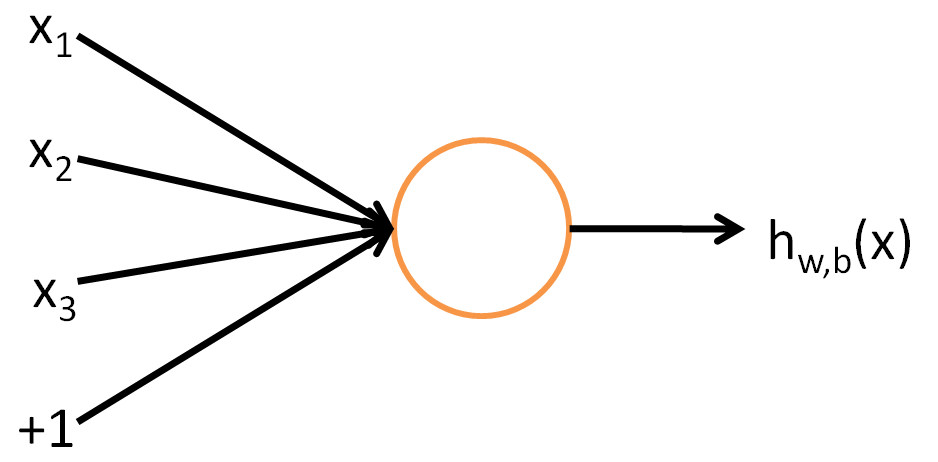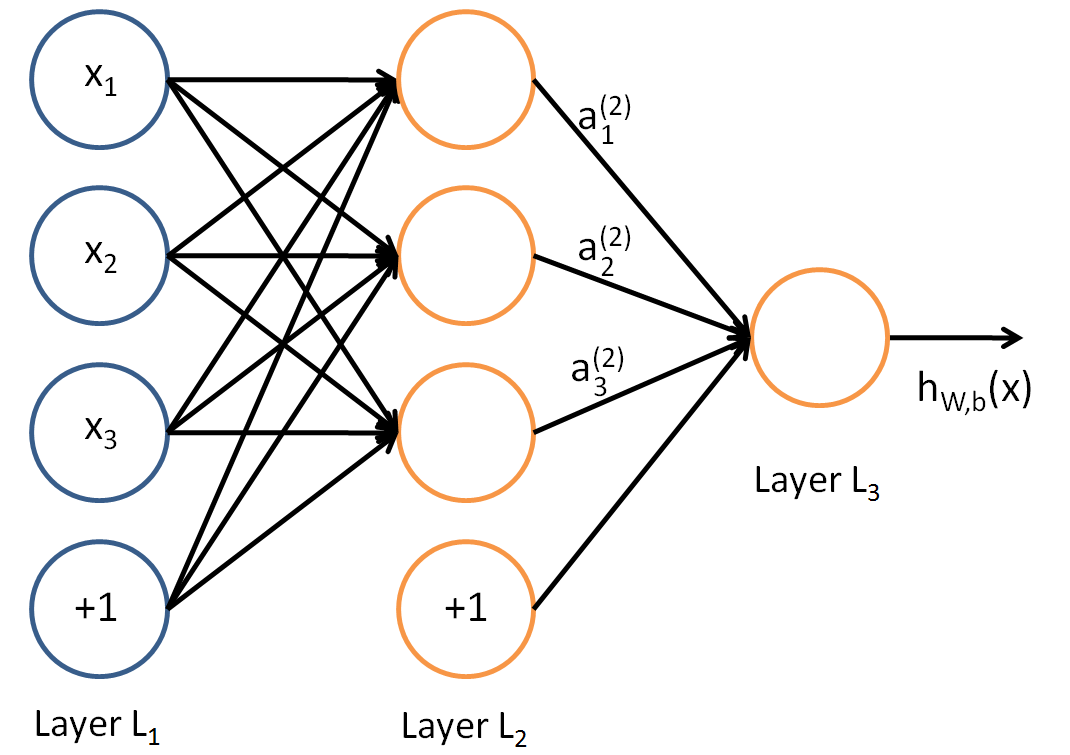Artificial Neural Networks (ANNs) have totally changed what computers are capable of learning. Though neural networks date back 1940s, we are seeing an astonishing amount of increase of its applications in the recent 5–10 years.

Artificial neural networks are modeled on the functioning of the human brain, where the input is converted into output based on a series of transformations. Though they are capable of achieving complex tasks, the way they work is fairly straight forward.

Three main concepts which explain the working of neural networks:

1. Neuron
2. Feed forward network
3. Back propagation

## Neuron

This is a simple computation unit which takes a single or multiple inputs and spits out an output. The function here, transforming the input to output is generally a simple logistic function. This is called an activation function.## Feed forward network

Now that we know how a single neuron works, lets see how a simple neural network looks like.We create a network where each layer contains some number of neurons. The outputs of neurons in layer L1 are inputs to the neurons in layer L2, outputs of neurons in layer L2 are inputs to the neurons in layer L3 and so on. In the final layer, we can have a single neuron which takes input from the previous layer and outputs a value. The input to any layer is not just the output of the previous layer, rather it is the weighted output which goes as input to the next layer (these are the connections). Layer L1 is called the *input layer*, L3 is the *output layer* and L2 is the *hidden layer.* We can have more than one hidden layer.

Basically, as the name suggests, we are feeding forward the outputs of a layer to the layer in front as inputs.

This entire calculation from a high level can be thought of as an inception of functions. Assuming we have 4 layers in total:

• f(.) is the function for outputs in L2
• g(.) is the function for outputs in L3
• h(.) is the function for outputs in L4 (the last layer)

then the output is nothing but *h(g(f(x)))*.

Now that we know how the feed forward network works, lets try to understand the learning process.

First, we feed the input layer with the inputs and assign random weights to them. The product of weights and inputs now become the input to L2 and the same is done to the subsequent layers. Finally, we get a value at the output layer which completes one forward pass.

Now we compare the computed output with the actual output (target). There will be a difference between the actual and computed value, which is nothing but the error.

If we think about the reason for the error, it is due to the weights we randomly assigned. It is not the right weights we need to use. The error can be minimized by changing the weight in the previous layer. Now we adjust the weights in L3 to correct the error. Changing weights in L3 means, we need to adjust the weights in L2 to accommodate the change of weights in L3 and the same process continues till the input layer. In other words, we are propagating the error back to the input layer by changing the weights.

### This process of adjusting the weights in each layer to correct the error is called Back propagation.

Initially, the working of back propagation might seem to be complex. But in its core it is nothing but simple derivatives.

References